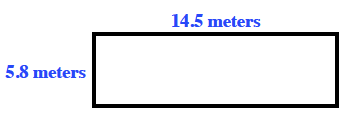### Home > ACC6 > Chapter 6 Unit 6 > Lesson CC1: 6.1.4 > Problem6-53

6-53.

Find the area and perimeter of a rectangle that is $14.5$ meters by $5.8$ meters.

Below is a sketch of the rectangle described in this problem.
Use this diagram if it helps you with finding the area and the perimeter.Remember that area is the space within a shape and perimeter is the distance around a shape.